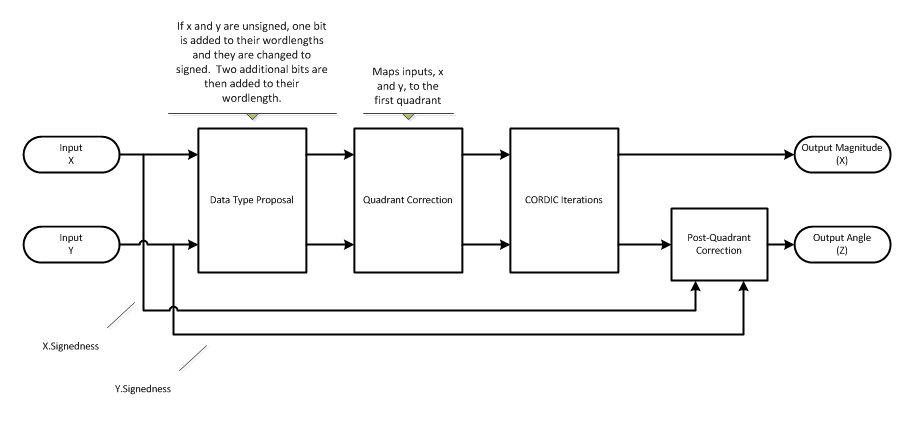# cordicangle

CORDIC-based phase angle

## Syntax

``theta = cordicangle(c)``
``theta = cordicangle(c,niters)``

## Description

example

````theta = cordicangle(c)` returns the phase angle in the interval `[-π,π]` for each element of a complex array `c`.```
````theta = cordicangle(c,niters)` performs `niters` iterations of the CORDIC algorithm.```

## Examples

collapse all

Use the `cordicangle` function to compute the CORDIC-based phase angle for double-precision and fixed-point inputs.

```dblRandomVals = complex(rand(5,4),rand(5,4)); theta_dbl_ref = angle(dblRandomVals); theta_dbl_cdc = cordicangle(dblRandomVals)```
```theta_dbl_cdc = 5×4 0.6777 1.4428 1.3512 1.4002 0.0394 1.2122 0.0328 1.0252 1.4223 0.6222 0.2816 0.3334 0.7966 0.6003 0.0948 0.8758 0.8208 0.1756 0.1208 0.0359 ```
```fxpRandomVals = fi(dblRandomVals); theta_fxp_cdc = cordicangle(fxpRandomVals)```
```theta_fxp_cdc = 0.6777 1.4426 1.3513 1.4001 0.0393 1.2122 0.0327 1.0254 1.4224 0.6223 0.2817 0.3333 0.7964 0.6003 0.0950 0.8757 0.8208 0.1758 0.1208 0.0359 DataTypeMode: Fixed-point: binary point scaling Signedness: Signed WordLength: 16 FractionLength: 13 ```

## Input Arguments

collapse all

Input array, specified as a scalar, vector, matrix, or multidimensional array. When the elements of `c` are nonnegative real numbers, `cordicangle` returns `0`. When the elements of `c` are negative real numbers, `cordicangle` returns `π`.

Data Types: `single` | `double` | `fi`
Complex Number Support: Yes

Number of iterations the CORDIC algorithm performs, specified as a positive integer-valued scalar.

Increasing the number of iterations can produce more accurate results but also increases the expense of the computation and adds latency.

If you do not specify `niters`, or if you specify it as empty or nonfinite, the maximum allowed value is used. For fixed-point input, the maximum number of iterations is one less than the word length of `c`. For double-precision input, the maximum value is 52. For single-precision input, the maximum value is 23.

Data Types: `single` | `double` | `int8` | `int16` | `int32` | `int64` | `uint8` | `uint16` | `uint32` | `uint64` | `fi`

## Output Arguments

collapse all

Phase angle in radians, returned as a scalar, vector, matrix, or multidimensional array.

If `c` is floating-point, then `theta` has the same data type as `c`. Otherwise, `theta` has a fixed-point data type with the same word length as `c` and with a best-precision fraction length.

collapse all

### CORDIC

CORDIC is an acronym for COordinate Rotation DIgital Computer. The Givens rotation-based CORDIC algorithm is one of the most hardware-efficient algorithms available because it requires only iterative shift-add operations (see References). The CORDIC algorithm eliminates the need for explicit multipliers. Using CORDIC, you can calculate various functions such as sine, cosine, arcsine, arccosine, arctangent, and vector magnitude. You can also use this algorithm for divide, square root, hyperbolic, and logarithmic functions.

Increasing the number of CORDIC iterations can produce more accurate results but also increases the expense of the computation and adds latency.

## Algorithms

collapse all

### Signal Flow DiagramsCORDIC Vectoring KernelThe accuracy of the CORDIC kernel depends on the choice of initial values for X, Y, and Z. This algorithm uses the following initial values:

### fimath Propagation Rules

CORDIC functions discard any local `fimath` attached to the input.

The CORDIC functions use their own internal `fimath` when performing calculations:

• `OverflowAction``Wrap`

• `RoundingMethod``Floor`

The output has no attached `fimath`.

 Volder, Jack E. “The CORDIC Trigonometric Computing Technique.” IRE Transactions on Electronic Computers. EC-8, no. 3 (Sept. 1959): 330–334.

 Andraka, Ray. “A Survey of CORDIC Algorithm for FPGA Based Computers.” In Proceedings of the 1998 ACM/SIGDA Sixth International Symposium on Field Programmable Gate Arrays, 191-200. https://dl.acm.org/doi/10.1145/275107.275139.

 Walther, J.S. “A Unified Algorithm for Elementary Functions.” In Proceedings of the May 18-20, 1971 Spring Joint Computer Conference, 379-386. https://dl.acm.org/doi/10.1145/1478786.1478840.

 Schelin, Charles W. “Calculator Function Approximation.” The American Mathematical Monthly, no. 5 (May 1983): 317-325. https://doi.org/10.2307/2975781.